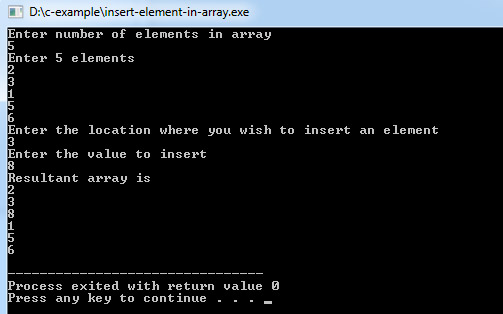## C Programming Examples Tutorial Index

This C program code will insert an element into an array, and it does not mean increasing size of the array.

For example consider an array n having four elements:
n = 1, n = 2, n = 3 and n = 4
And suppose you want to insert a new value 60 at first position of array. i.e. n = 60, so we have to move elements one step below so after insertion
n = 1 which was n initially, n = 2, n = 3 and n = 4.

Program:

``````#include <stdio.h>

int main()
{
int array, position, c, n, value;

printf("Enter number of elements in the array\n");
scanf("%d", &n);

printf("Enter %d elements\n", n);

for (c = 0; c < n; c++)
scanf("%d", &array[c]);

printf("Please enter the location where you want to insert an new element\n");
scanf("%d", &position);

scanf("%d", &value);

for (c = n - 1; c >= position - 1; c--)
array[c+1] = array[c];

array[position-1] = value;

printf("Resultant array is\n");

for (c = 0; c <= n; c++)
printf("%d\n", array[c]);

return 0;
}``````

Program Output: